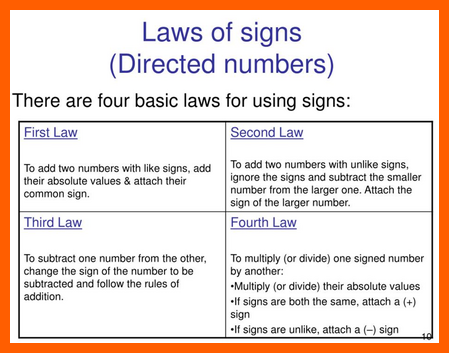# Caesar’s Hors d’oeuvres--Why Our Students Fail in Mathematics? Two Principles```Hors d'oeuvres # 011
```

Why Our Students --When Problem Solving--Fail in Mathematics? Two Principles!

SIMPLE: They do not know it! Why?

1. The Law of the Signs:

• + x + = + ( positive x positive = positive) [First Law]
• + x  - = -  ( positive x negative = negative) [Second Law]
• x  + = -  ( negative x positive = negative) [Third Law]
• x  - = +  ( negative x negative = positive[Fourth Law]2. Please Excuse My Dear Aunt Sally [PEMDAS].

Just  make a follow-up step-by step  and arithmetically solve each itemized task of this sequence:

• Parenthesis
• Exponent
• Multiplicationn
• Division